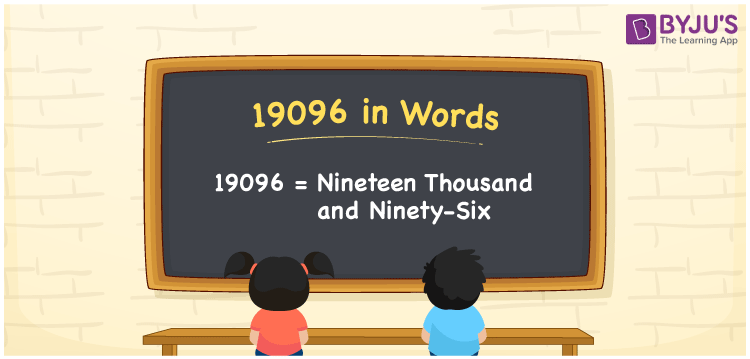# 19096 in Words

We can write 19096 in words as Nineteen thousand and ninety-six. For instance, the price of a dishwasher is Rs. 19096; we can communicate this statement as “The price of a dishwasher is Nineteen thousand and ninety-six rupees”. In this article, you will learn how to write the number 19096 in words using a place value chart using a simple technique, along with some interesting facts about 19096.

 19096 in words Nineteen thousand and ninety-six Nineteen thousand and ninety-six in Numbers 19096

## 19096 in English Words

Generally, we use the English alphabet to express numbers in words. So, we spell 19096 in English words as “Nineteen thousand and ninety-six”.## How to Write 19096 in Words?

Let’s understand how to write the number 19096 in words with the help of a place value chart, which is given below.

 Ten thousand Thousands Hundreds Tens Ones 1 9 0 9 6

Here, ones = 6, tens = 9, hundreds = 0, thousands = 9, ten thousand = 1

The above numbers can be written as:

1 × Ten thousand + 9 × Thousand + 0 × Hundred + 9 × Ten + 6 × One

= 1 × 10000 + 9 × 1000 + 0 × 100 + 9 × 10 + 6 × 1

= 10000 + 9000 + 90 + 6

= Ten Thousand + Nine thousand + Ninety + Six

= Nineteen thousand and ninety-six

Therefore, 19096 in words = Nineteen thousand and ninety-six.

As we know, 19096 is a natural number that is the successor of 19095 and predecessor of 19097.

19096 in words – Nineteen thousand and ninety-six

Is 19096 an odd number? – No

Is 19096 an even number? – Yes

Is 19096 a prime number? – No

Is 19096 a composite number? – Yes

Is 19096 a perfect square number? – No

Is 19096 a perfect cube number? – No

## Frequently Asked Questions on 19096 in Words

Q1

### Write 19096 in words.

We can write the number 19096 in words as Nineteen thousand and ninety-six.
Q2

### How to write Rs. 19096 in words on a cheque?

On a cheque, we generally express Rs. 19096 in words as “Nineteen thousand and ninety-six rupees only”.
Q3

### What is the number name of 19096?

The number name of 19096 is given by Nineteen thousand and ninety-six.